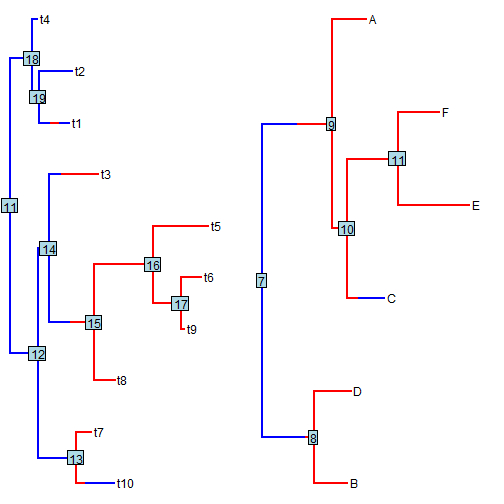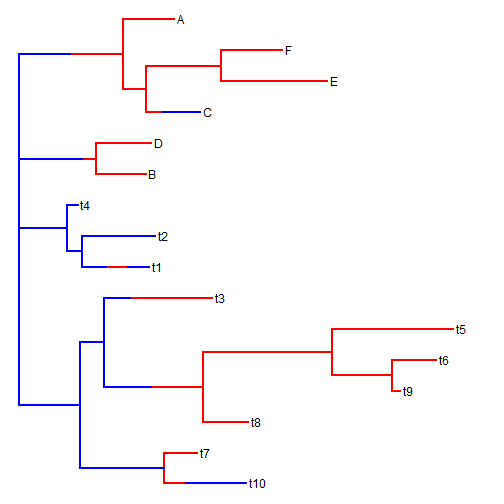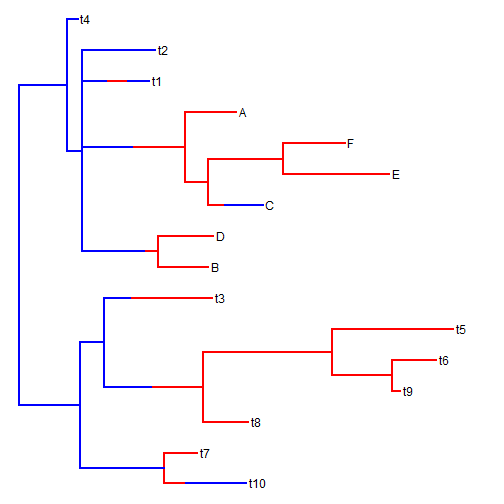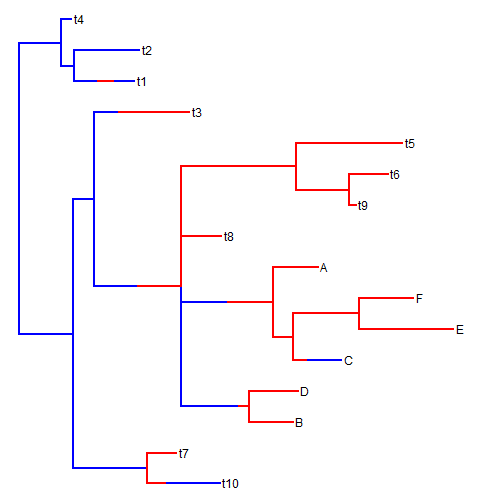## Thursday, May 19, 2016

### `bind.tree` for objects of class `"simmap"` (a preliminary version)

A R-sig-phylo member phytools user posted the following question yesterday:

“Is it possible to bind two simmaps together? When I attempt to run the following code, the call to bind.tree doesn't throw any errors but I get an error when I attempt to plot the resulting tree.”

Unfortunately, at present the answer is no; however on my flight this afternoon I quickly hacked together the following function which wraps around the ape function `bind.tree`. This function does bind two objects of class `"simmap"`; however it does not permit the donor tree to have a root edge, and the subtree to be added cannot be bound along an edge. If I can add those features later, then perhaps I will include this function with phytools.

``````bind.tree.simmap<-function(x,y,where="root"){
x<-reorder(x)
y<-reorder(y)
rootx<-x\$edge[1,1]
rooty<-y\$edge[1,1]
Mx<-rbind(matchLabels(x,xy),matchNodes(x,xy,"distances"))
My<-rbind(matchLabels(y,xy),matchNodes(y,xy,"distances"))
xy\$maps<-vector(mode="list",length=nrow(xy\$edge))
ix<-sapply(Mx[-which(Mx[,1]==rootx),1],
function(x,y) which(y==x),y=x\$edge[,2])
ixy<-sapply(Mx[-which(Mx[,1]==rootx),2],
function(x,y) which(y==x),y=xy\$edge[,2])
xy\$maps[ixy]<-x\$maps[ix]
iy<-sapply(My[-which(My[,1]==rooty),1],
function(x,y) which(y==x),y=y\$edge[,2])
ixy<-sapply(My[-which(My[,1]==rooty),2],
function(x,y) which(y==x),y=xy\$edge[,2])
xy\$maps[ixy]<-y\$maps[iy]
xy\$mapped.edge<-phytools:::makeMappedEdge(xy\$edge,xy\$maps)
ns<-c(setNames(getStates(xy,"tips"),1:Ntip(xy)),
getStates(xy,"nodes"))
xy\$node.states<-cbind(ns[as.character(xy\$edge[,1])],
ns[as.character(xy\$edge[,2])])
xy\$states<-getStates(xy,"tips")
attr(xy,"class")<-c("simmap",class(xy))
xy
}
``````

Basically, the way it works is by (1) first binding the two trees together as an object of class `"phylo"`, then (2) goes back and matches the edges of each of the two input trees to the new tree, next (3) it copies the mappings from the input trees to the bound tree, finally (4) it creates all the other components and attributes of the new object of class `"simmap"` that has been created.

It works, so far as I can tell. For instance:

``````colors<-setNames(c("blue","red"),letters[1:2])
colors
``````
``````##      a      b
## "blue"  "red"
``````
``````t1
``````
``````##
## Phylogenetic tree with 10 tips and 9 internal nodes.
##
## Tip labels:
##  t10, t7, t8, t9, t6, t5, ...
##
## The tree includes a mapped, 2-state discrete character with states:
##  a, b
##
## Rooted; includes branch lengths.
``````
``````t2
``````
``````##
## Phylogenetic tree with 6 tips and 5 internal nodes.
##
## Tip labels:
##  "B" "D" "C" "E" "F" "A"
##
## The tree includes a mapped, 2-state discrete character with states:
##  a, b
##
## Rooted; includes branch lengths.
``````
``````x11()
par(mfcol=c(1,2))
plot(t1,colors); nodelabels()
plot(t2,colors); nodelabels()
``````First, let's bind them together at the root (the default):

``````t3<-bind.tree.simmap(t1,t2)
plot(t3,colors)
``````Next, at some internal node - say node 19:

``````t4<-bind.tree.simmap(t1,t2,where=19)
plot(t4,colors)
``````Of course, pasting two trees together means that you could see a change in character state precisely at a node (something that cannot happen with stochastic mapping):

``````t5<-bind.tree.simmap(t1,t2,where=15)
plot(t5,colors,split.vertical=TRUE)
``````The trees for this example were simulated as follows:

``````Q<-matrix(c(-1,1,1,-1),2,2)
rownames(Q)<-colnames(Q)<-letters[1:2]
t1<-sim.history(rtree(n=10),Q)
``````
``````## Done simulation(s).
``````
``````t2<-sim.history(rtree(n=6,tip.label=LETTERS[1:6]),Q)
``````
``````## Done simulation(s).
``````